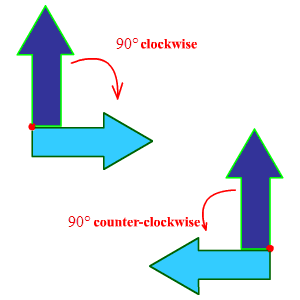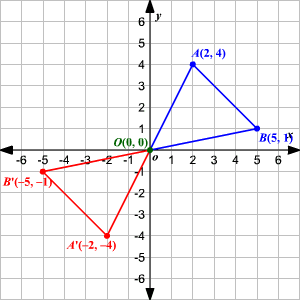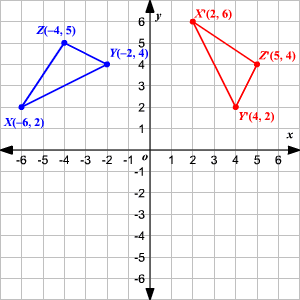# Angle of Rotation

A rotation is a transformation in a plane that turns every point of a figure through a specified angle and direction about a fixed point.

The fixed point is called the center of rotation .

The amount of rotation is called the angle of rotation and it is measured in degrees.You can use a protractor to measure the specified angle counterclockwise.

Consider the figure below.Here, $\Delta A\text{'}B\text{'}O$ is obtained by rotating $\Delta ABO$ by $180°$ about the origin. Observe that both $AOA\text{'}$ and $BOB\text{'}$ are straight lines.

So, $m\angle AOA\text{'}=180°=m\angle BOB\text{'}$ .

Example:How many degrees has the $\Delta XYZ$ been rotated counterclockwise to obtain the $\Delta X\text{'}Y\text{'}Z\text{'}$ ?

$\begin{array}{l}\text{A}.\text{\hspace{0.17em}}\text{\hspace{0.17em}}90°\\ \text{B}.\text{\hspace{0.17em}}\text{\hspace{0.17em}}180°\\ \text{C}.\text{\hspace{0.17em}}\text{\hspace{0.17em}}270°\\ \text{D}.\text{\hspace{0.17em}}\text{\hspace{0.17em}}360°\end{array}$

Identify the corresponding vertices of the rotation.

$\begin{array}{l}X\left(-6,2\right)\to X\text{'}\left(2,6\right)\\ Y\left(-2,4\right)\to Y\text{'}\left(4,2\right)\\ Z\left(-4,5\right)\to Z\text{'}\left(5,4\right)\end{array}$

The point of rotation is the origin, draw lines joining one of the points, say $X$ and it's image to the origin.

You can see that the lines form an angle of $270°$ , in the counterclockwise direction.

Therefore, $\Delta X\text{'}Y\text{'}Z\text{'}$ is obtained by rotating $\Delta XYZ$ counterclockwise by $270°$ about the origin.

So, the correct choice is $\text{C}$ .

Also note that the relation between the corresponding vertices is $\left(x,y\right)\to \left(-y,x\right)$ which shows a counterclockwise rotation of $270°$ about the origin.